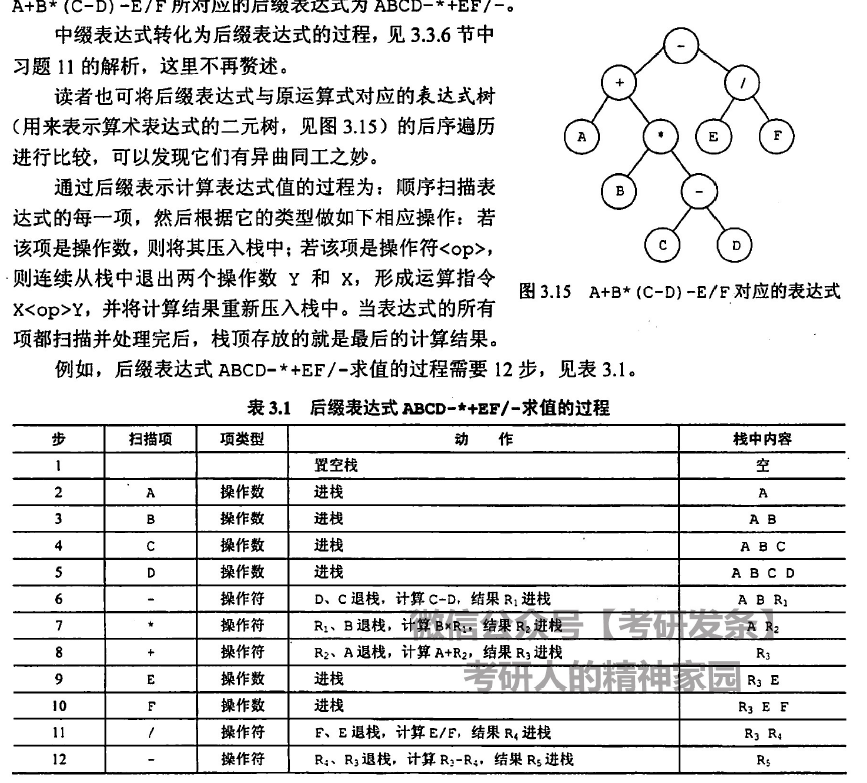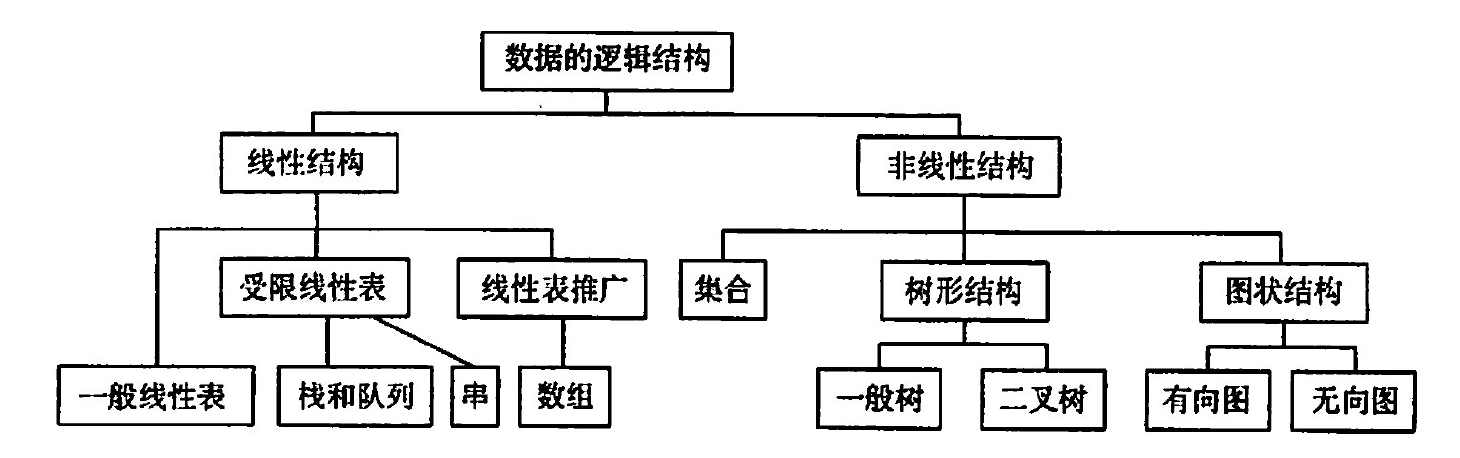## Some of watch

### 栈、队列和数组### 树

• 结点数=度数+1
• 度为m的树第i层最多有$m^{i-1}$个节点
• 高为h的m叉树最多有$(m^h-1)/(m-1)$（实际上就是等比数列前n项和）

• $n=n_0+n_1+n_2=n_1+2n_2+1 \rightarrow n_0=n_2+1$
• 非空二叉树的第k蹭上至多有$2^{k-1}$结点
• 高度为h的二叉树最多有$2^h-1$### 图

Kruskal 找权值最小边逐渐加入（适用于点多） $O(ElogE)$

Floyd 求各顶点最短路径，表格比较复杂 $O(V^3)$

ve 事件最早发生时间，正向
vl 事件最迟发生时间，倒推
e 活动最早发生时间，正退
l 活动最迟发生时间，vl-活动时间
d=l-e 最迟开始时间

### 查找

B树 也不容易
B+树

B树n叉就有被n-1和关键字分为n个范围。为了使得查找和存储效率较高，一般我们通过认为m叉查找树中除根节点外至少有[m/2]个分叉，即m/2-1个关键字，且需要所有子树高度相同

n个关键字必有n+1个叶子节点

B+树相较于B树，把区间换成了关键字。B+树支持顺序查找(子节点全部由链表连接)，也可以通过树查找，非叶子节点都仅做索引，且为叶子节点中的最大值。

### 排序B树 也不容易
B+树